# 第七章：排序

## 1.排序的基本概念

排序 重新排列表中的元素,使表中的元素满足按关键字递增或递减

排序算法的稳定性 若待排序表中有两个元素RiRj,其对应的关键字ki=kj,且在排序前RiRj前面，若使用某排序算法后,Ri仍然在Rj前面。则称这个排序算法是稳定的,否则称排序算法不稳定

内部排序 指在排序期间元素全部存放在内存中的排序

外部排序 指在排序期间元素无法全部同时存放在内存中，必须在排序的过程中根据要求不断地在内、外存之间进行移动‘

## 2.插入排序

### 2.1.直接插入排序

插入排序 每次将一个待排序的序列插入到一个前面已排好序的子序列当中

直接插入排序 空间复杂度O(1)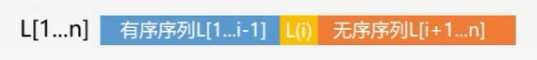• 初始L是一个已经排好序的子序列
• 对于元素L(i)(L(2)~L(n))插入到前面已经排好序的子序列当中：
• 1)查找出L(i)在L[1....i-1]中的插入位置k
• 2)将L[k....i-1]中的所有元素全部后移一个位置
• 3)将L(i)复制到L(k)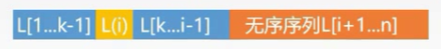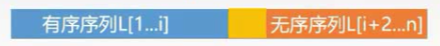//注意我们数组下标为0的位置不存储值
//注意下面的排序结果得到的是一个递增的序列
void InsertSort(ElemType A[],int n){
int i,j;
for(i=2;i<=n;i++){//从下标为2的元素开始，因为下标为1的元素为1个，我们设为已经排好序
A=A[i]; //A位置相当于一个哨兵，暂存待插入的元素
//如果当前判断的这个值小于有序序列中的一个值,向后移动一个位置
//这里的循环j--可以发现是从后往前进行比较
//这里的A还有一个好处就是防止j--变成-1，因为当j减到0的时候它的值一定的等于A.key
for(j=i-1;A.key<A[j].key;j--){
A[j+1]=A[j]; //将值向后移动一个位置
}
A[j+1]=A;//修改位置上的值
}
}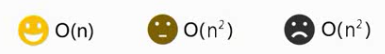直接插入排序是稳定的！ 因为我们判断是否移动是小于号，而等于的情况我们是不移动的，值得相对位置不变。它适用于链式存储和顺序存储。

### 2.2.折半插入排序

void BInsertSort(ElemType A[],int n)//n是数组大小
{
int i,j;
int low,high,mid;//折半查找辅助变量
for(i=2;i<=n;i++){
A=A[i];//同样A位置相当于一个哨兵，暂存待插入的元素
//折半查找开始
low=1;high=i-1;
while(low<=high){
mid=(low+high)/2;
if(A[mid].key>A.key){
high=mid-1;
}else{
low=mid+1;
}
}
//折半查找结束
//元素移动开始
for(j=i-1;j>=high+1;j--){
A[j+1]=A[j];
}
//元素移动结束
A[high+1]=A;//赋值
}
}


### 2.3.希尔排序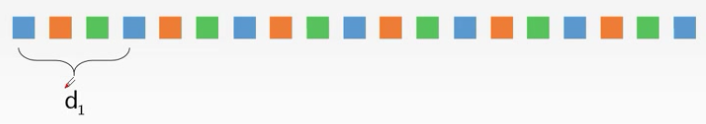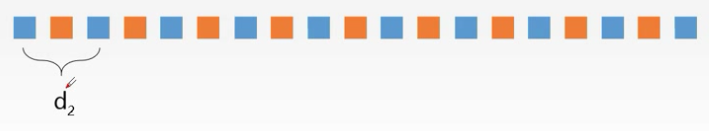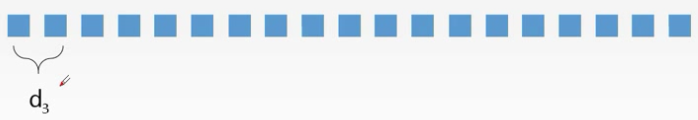void ShellSort(ElemType A[],int n){
for(int dk=n/2;dk>=1;dk=dk/2){
for(int i=dk+1;i<=n;++i){
if(A[i].key<A[i-dk].key){
A=A[i];
for(int j=i-dk;j>0&&A.key<A[j].key;j-=dk){
A[j+dk]=A[j];
}
A[j+dk]=A;
}
}
}
}


## 3.交换排序

### 3.1.冒泡排序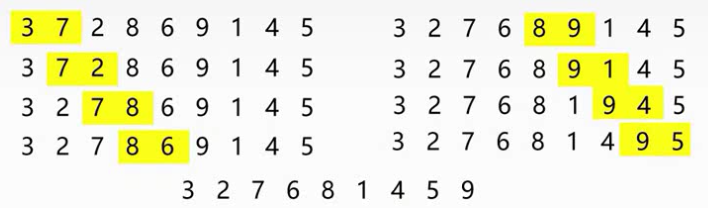void BubbleSort(ElemType A[],int n){
for(int i=0;i<n-1;i++){//n-1次冒泡
bool flag=false; //初始化为false
//两两比较的一个过程，这里是从后往前的比较两个相邻元素，每一次冒泡将最小值移动到最前面
for(int j=n-1;j>i;j--){
if(A[j-1].key>A[j].key){//如果前面的元素大于后面的元素
swap(A[j-1],A[j]);//交换两个元素的值
flag=true;//赋值为true 表示存在逆序的序列
}
}
//这里如果flag为false说明if判断没进入，说明当前的序列已经排好序列了，可以减少冒泡的次序
if(flag==false){
return;//终止排序
}
}
}


### 3.2.快速排序Partition的基本思路：

1. high向前移动找到第一个比pivot小的元素

2. low向后移动找到第一个比pivot大的元素

3. 交换当前两个位置的元素

4. 继续移动标记，执行 1、2、3 的过程，直到low大于等于high为止

Partition代码实现：

//这里代码返回整型，是因为我们要返回划分的元素的位置的下标，我们就可以通过这个位置将序列划为两部分
//传参：数组，数组的第一个元素下标，最后个元素的下标
int Partition(ElemType A[],int low,int high){
ElemType pivot=A[low];//默认使用第一个元素的作为基准
while(low<high){//一旦low等于high，代表重合划分完毕
//向前移动找到第一个小于pivot的值
while(low<high && A[high]>=pivot){
high--;//向前移动
}
//将找到的这个值赋值low，注意我们第一步已经将pivot的值保存到了pivot中，所以low下标的值不会丢失
A[low]=A[high];//这里也A[low]也充当了赋值变量暂存A[high]的值
//向后移动找到第一个大于pivot的值
while(low<high && A[low]<=pivot){
low++;
}
//将找到的这个元素赋值给high
A[high]=A[low];
//到这里相当于交换了两个元素值
}
//循环结束相当于low==high
A[low]=pivot;//然后将pivot的值赋值给A[low]
return low;//接着将low基准的位置返回
}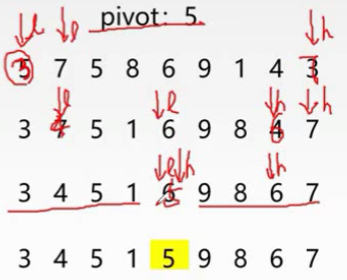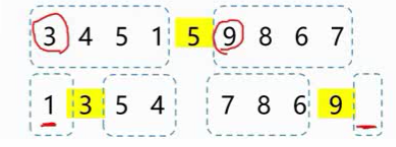//采用递归的方式调用QuickSort，每次判断是否进行Partition
void QuickSort(ElemType A[],int low,int high){
if(low<high){
int pivotpos=Partition(A,low,high);//获取基准
QuickSort(A,low,pivotpos-1);//数组前面部分
QuickSort(A,pivotpos+1,high);//数组后面部分
}
}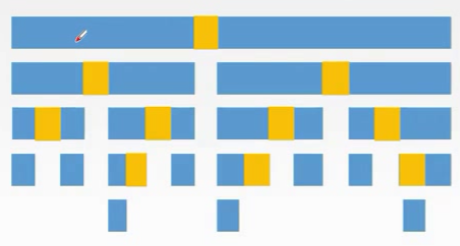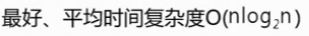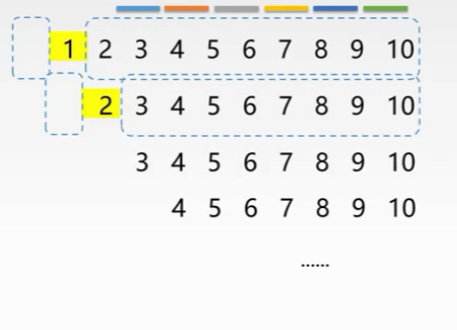## 4.选择排序

### 4.1.简单选择排序//循环找比当前最小值后面更小的值进行交换
void SelectSort(ElemType A[],int n){
for(int i=0;i<n-1;i++){
int min=i;
for(int j=i+1;j<n;j++){
if(A[j]<A[min]){
min=j;
}
}
if(min!=i){
swap(A[i],A[min]);
}
}
}


### 4.2.堆排序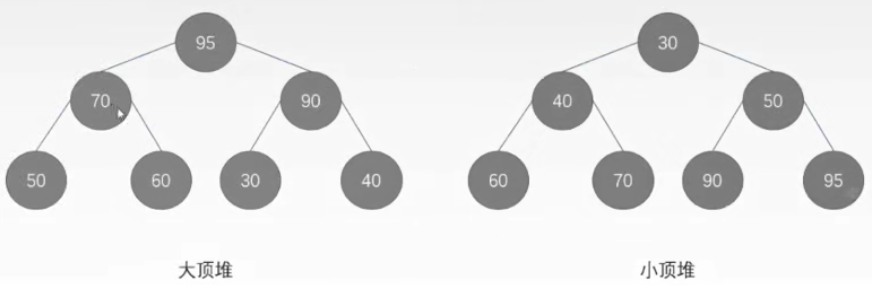12 52 19 45 23 45 92 （以大顶堆排序为例），首先排成一个完全二叉树序列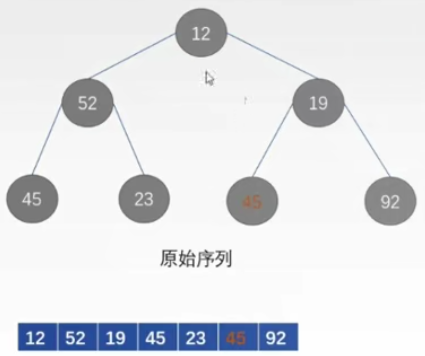①建堆对初始序列的完全二叉树调整成一个大顶堆调整方法：二叉树由下至上由右至左(数组的下标由大到小)。检查每个结点是否满足大顶堆的要求,如果不满足进行调整。

• 45 23 45 92 四个结点都是叶子结点,不需要调整

• 19<45<92，所以要用92和19进行交换

• 52>45且>23 不需要调整

• 12<52<92 要用92交换12

• 12<19<45 要用45和12交换

• 到这里就建好了一个大顶堆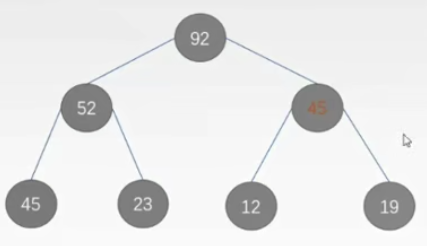②将堆顶结点和最后一个结点19交换，也就是将最大值92移动到数组的最末尾，有序序列中加入了结点92，无序序列减少结点92，到这里堆排序的第一趟完成。【此时二叉树不满足堆的性质了，需要调堆】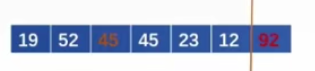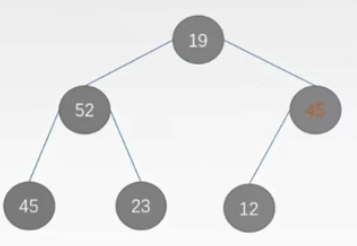③调堆：调整二叉树的结点使得满足大顶堆的要求。调整方法和建堆时一样。

• 45>12不需要调整，52也不需要
• 19<45<52需要将结点19和52交换
• 19<23<45需要将结点19和45交换
• 到这里又调成了一个大顶堆类似之前的操作，选出根结点52作为有序序列的第二个数值

①当i>1时，结点i的双亲结点编号为i/2，即当i为偶数时，其双亲结点的编号为i/2。它是双亲结点的左孩子，当i为奇数时，其双亲结点的编号为(i-1)/2，它是双亲结点的右孩子。

②当2i≤N时，结点i的左孩子编号为2i，否则无左孩子。

③当2i+1≤N时，结点i的右孩子编号为2i+1，否则无右孩子。

//建堆代码
void BuildMaxHeap(ElemType A[],int len){
//由数组下标高出往低处，从第一个可能需要调整的非叶结点
//开始检查，直到根结点（注意根节点下标不是0，是从1开始存储）
for(int i=len/2;i>0;i--){
}
}

//调堆代码
//A是存储堆的数组，k是需要检查的结点下标，len是堆中结点的个数
//A暂存这个需要检查的结点值
A=A[k];
//从这个结点的左孩子（2*k）开始往下比较
for(int i=2*k;i<=len;i*=2){
//如果发生交换，对交换过的结点继续和它的孩子比较
//判断左右孩子谁更大，如果右孩子大一些，就只要考虑和右孩子比较（i++）
if(i<len&&A[i]<A[i+1]){
i++;//i++ 指向右孩子
}
//如果这个电的值不小于它的较大的孩子结点的值，则不需要交换
if(A>=A[i]){
break;
}else{
//如果这个结点值小于他较大孩子
//结点值则将较大的孩子节点值赋值给该结点
A[k]=A[i];
//i 赋值给 k 也就是从 i 开始继续往下检查
//注意我们这里是 i*=2，就是从每个待检查的结点的左孩子开始
//比较，直到所有结点检查结束
k=i;
}
}
//检查到最后k的值就是最后一轮交换过的结点位置下标，将该节点换过去
A[k]=A;
}

//堆排序完整代码
void HeapSort(ElemType A[],int len){
BuildMaxHeap(A,len);//初始建堆，得到第一个堆顶元素
for(int i=len;i>1;i--){//n-1趟的交换和建堆过程
//输出堆顶元素（和堆底元素交换）
ElemType temp=A[i];
A[i]=A;
A=temp;
printf(A[i]);
}
}


## 5.归并排序

①首先将整个序列的每个关键字看成一个单独的有序的子序列

②两两归并,49和38归并成{38 49},65和97归并成(65 97,}76和13归并成(13 76}，27没有归并对象

③两两归并,{38 49}和{65 97归并成{38 49 65 97}，{13 76}和27归并成{13 27 76}

④两两归并,{38 49 65 97}和{13 27 76}归并成{13 27 38 49 65 76 97}

ElemType *B=(ElemType *)malloc((n+1)*sizeof(ElemType));//辅助数组B（这里采用动态分配内存）
//辅助数组的作用：首先将A中的数组全部复制到B中，然后在B中进行归并排序，将归并出的数据放回到A中的对应位置上，当归并结束后B数组不用管它,A数组就是排好序的数组

//归并代码
//参数：待归并数组A，第一段首位下标，第一段末位下标，第二段的末位下标
//注意：我们这里归并的这两段都是有序的（如何有序不重要，我们这里主要分析归并的过程）
void Merge(ElemType A[],int low,int mid,int high){
//表A的两段A[low....mid]和A[mid+1....high]各自有序，将他们合并成一个有序表
for(int k=low;k<high;k++){//将A中所有元素复制到B中
B[k]=A[k];
}
//i 和 j 初始值分别指向两段的首位下标，k是归并之后复制到A数组的下标计数器
for(int i=low,j=mid+1,k=i;i<mid&&j<=high;k++){
if(B[i]<=B[j]){//比较B的左右两段中的元素，将较小的复制到A中
A[k]=B[i++];
}else{
A[k]=B[j++];
}
}
//因为可能存在未归并完的情况，未归并完说明剩下的这一段，肯定全部
//大于前面的值，所以直接赋值过来即可
while(i<=mid){
A[k++]=B[i++];//若第一个表未检测完，直接将剩下的部分赋值过来
}
while(j<=high){
A[k++]=B[j++];//若第二个表未检测完，直接将剩下的部分赋值过来
}
}

//归并排序代码
void MergeSort(ElemType A[],int low,int high){
if(low<high){
int mid=(low+high)/2;//从中间划分未两个子序列
MergeSort(A,low,mid);//对左侧子序列进行递归排序
MergeSort(A,mid+1,high);//对右侧子序列进行队规排序
Merge(A,low,mid,high);//归并
}
}


Merge第①趟:子序列长度为2.所以有n/2对子序列,每个子序列需要执行1次 Merge 。Merge时间复杂度为子序列长度之和，即2。所以第①趟 merge的时间复杂度为(n/2*2)=0(n)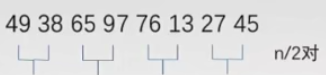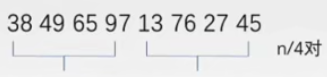**稳定性：**稳定的

## 6.基数排序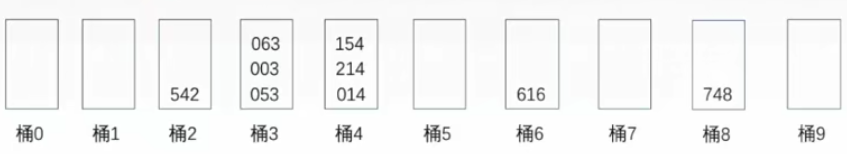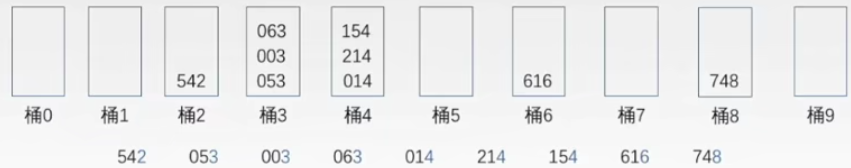003 014 214 616 542 748 154 053 063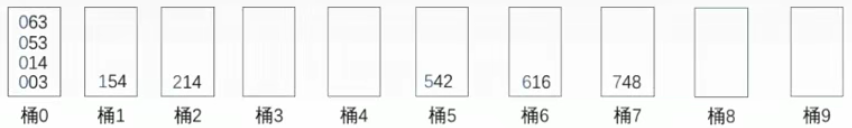003 014 053 063 154 214 542 616 748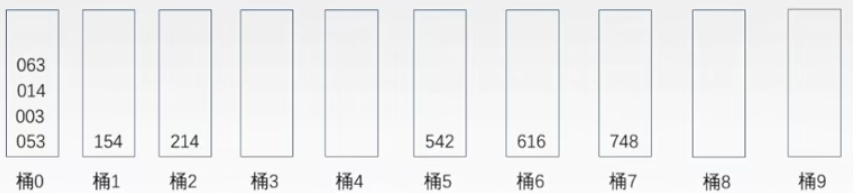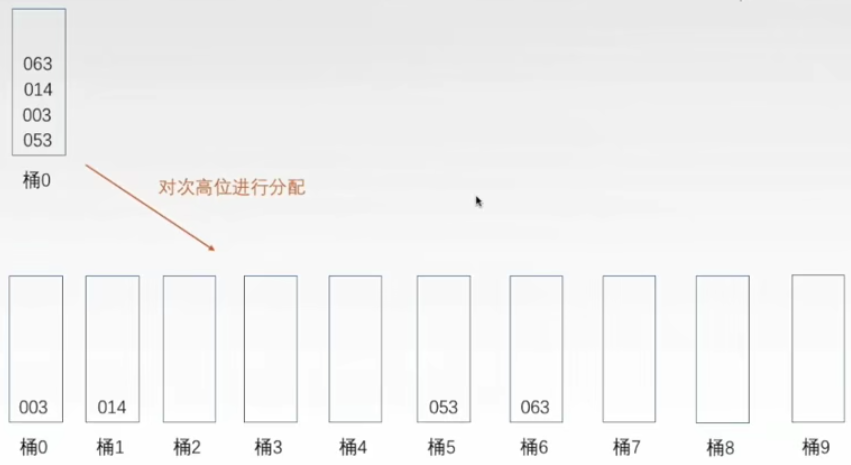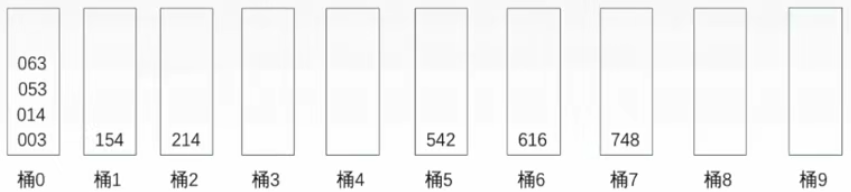## 7.理木客